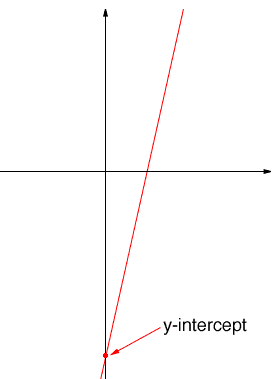Quandaries and Queries Hi, i would like to know if you can answer this question for me   Find the x and y intercept fo y = 8x - 5 thanks The y-intercept is the y-cordinate of the point where the graph crosses the y-axis. For example in the diagram below the red dot marks the place where the graph crosses the y-axis.Since this point is on the y-axis its coordinates ate (0,b) for som number b. This point is on the line y = 8x - 5 Thus b = 80 - 5 = -5 Thus b = -5. Hence the y-intercept is -5. Can you now find the x-intercept? Penny Go to Math Central